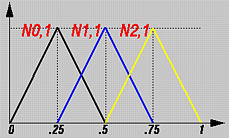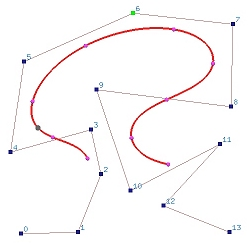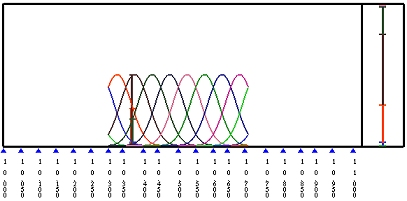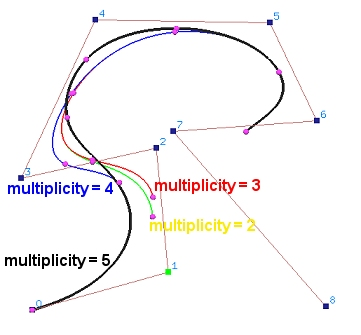# B-spline Curves: Open CurvesAs mentioned earlier, if the first and last knots do not have multiplicity p+1, where p is the degree of a B-spline curve, the curve will not be tangent to the first and last legs at the first and last control points, respectively. The curve is an open B-spline curve. In this case, we should be careful about one additional restriction. On a previous page we showed an example of basis function computation using knot vector U = { 0, 0.25, 0.5, 0.75, 1 }, where m = 4. If the basis functions are of degree 1 (i.e., p = 1), there are three basis functions N0,1(u), N1,1(u) and N2,1(u) as shown below.Since this knot vector is not clamped, the first and the last knot spans (i.e., [0, 0.25) and [0.75, 1)) have only one non-zero basis functions while the second and third knot spans (i.e., [0.25, 0.5) and [0.5, 0.75)) have two non-zero basis functions. Recall from the B-spline basis function important properties that on a knot span [ui, ui+1), there are at most p+1 degree p non-zero basis functions of degree p. Therefore, in this example, knot spans [0,0.25) and [0.75,1) do not have "full support" of basis functions. In general, for degree p, intervals [u0, up) and [um-p, um] will not have "full support" of basis functions and are ignored when a B-spline curve is open. Consequently, we have the following important note:

 For open B-spline curves, the domain is [up, um-p].

Consider a B-spline curve of degree 6 (i.e., p = 6) defined by 14 control points (i.e., n = 13). The number of knots is 21 (i.e., m = n + p + 1 = 20). If the knot vector is uniform, the knots are 0, 0.05, 0.10, 0.15, ..., 0.90, 0.95 and 1.0. The open curve is defined on [up, un-p] = [u6, u14] = [0.3, 0.7] and is not tangent to the first and last legs. The left figure below shows the curve and the right figure gives the B-spline basis functions.Even though some knot spans at both end are not used, the B-spline curve still is defined by all control points. As discussed on the B-spline basis functions page that there are at most p+1 basis functions Ni-p,p(u), Ni-p,p+1(u), ..., Ni,p(u) non-zero on knot span [ui, ui+1). Therefore, there are p+1 non-zero functions on [up, ui+1): N0,p(u), N1,p(u), ..., Np,p(u). This is a "full" count. Note that N0,p(u) has its tail in [up, up+1) and, hence, the contribution of control point P0 to the construction of the open B-spline curve is smaller than those of most other control points. A similar argument can be used to show that Pn is also involved in the definition of the curve.

Let us use an example to illustrate the change between an open curve and a clamped one. We start with an open B-spline curve of degree 4 defined by 9 control points (i.e., n = 8) and a uniform knot vector { 0, 1/13, 2/13, 3/13, ..., 12/13, 1 }. If we change the second knot 1/13 to 0 making 0 a double knot, the curve is the yellow one in the figure. In fact, this curve and the original one with 0 being a simple knot are almost identical. Now, if we change the third knot 2/13 to 0 making 0 a knot of multiplicity 3, the result is the red curve. If the fourth knot 3/13 is change to 0 (multiplicity 4), the resulting curve is the blue one. As you can see, these three open curves are not very different from each other. Now, let us make the fifth knot 4/13 to 0. Since 0 is now a knot of multiplicity 5 (i.e., p+1), the curve not only passes through the first control point but also is tangent to the first leg of the control polyline (i.e., clamped). As you can see from the figure, the shape of the curve changes drastically by pulling one end of it to the first control point. The same holds true if we make the last 5 knots to 1.